# Packages Required
library(ggplot2)  # Graphs
library(class)    # KNN
library(dummies)  # Dummy
library(gmodels)  # Cross Table

# Introduction

K-nearest neighbors is an extremely simple classification and regression algorithm that classifies or predicts a data point based on the majority vote of its nearest neighbors. The important characteristics of KNN are how many neighbors to consider (K) and the method used to calculate distance.

# The Heart Disease Data Set

This example uses the heart disease data set from Detrano (n.d.), retrieved from the UCI machine learning repository (Lichman, 2013), to investigate and implement an example of KNN classification by predicting the presence of heart disease using 13 features collected from 303 patients:

• age: age in years
• sex: sex (1 = male; 0 = female)
• cp: chest pain type
• Value 1: typical angina
• Value 2: atypical angina
• Value 3: non-anginal pain
• Value 4: asymptomatic
• trestbps: resting blood pressure (in mm Hg on admission to the hospital)
• chol: serum cholesterol in mg/dl
• fbs: (fasting blood sugar > 120 mg/dl) (1 = true; 0 = false)
• restecg: resting electrocardiograph results
• Value 0: normal
• Value 1: having ST-T wave abnormality (T wave inversions and/or ST elevation or depression of > 0.05 mV)
• Value 2: showing probable or definite left ventricular hypertrophy by Estes’ criteria
• thalach: maximum heart rate achieved
• exang: exercise induced angina (1 = yes; 0 = no)
• oldpeak = ST depression induced by exercise relative to rest
• slope: the slope of the peak exercise ST segment
• Value 1: up sloping
• Value 2: flat
• Value 3: down sloping
• ca: number of major vessels (0-3) colored by flourosopy
• thal: 3 = normal; 6 = fixed defect; 7 = reversible defect

The ‘num’ label defines the presence of heart disease with numbers 0-4: * 1-4 meaning presence of heart disease * 0 meaning no presence of heart disease

# Exploratory Data Analysis

The purpose of exploring the data first is to get familiar with it and to see if anything is of interest.

heart <- read.csv("processed.cleveland.data.csv") # Read in the data
str(heart)
## 'data.frame':    303 obs. of  14 variables:
##  $age : num 63 67 67 37 41 56 62 57 63 53 ... ##$ sex     : num  1 1 1 1 0 1 0 0 1 1 ...
##  $cp : num 1 4 4 3 2 2 4 4 4 4 ... ##$ trestbps: num  145 160 120 130 130 120 140 120 130 140 ...
##  $chol : num 233 286 229 250 204 236 268 354 254 203 ... ##$ fbs     : num  1 0 0 0 0 0 0 0 0 1 ...
##  $restecg : num 2 2 2 0 2 0 2 0 2 2 ... ##$ thalach : num  150 108 129 187 172 178 160 163 147 155 ...
##  $exang : num 0 1 1 0 0 0 0 1 0 1 ... ##$ oldpeak : num  2.3 1.5 2.6 3.5 1.4 0.8 3.6 0.6 1.4 3.1 ...
##  $slope : num 3 2 2 3 1 1 3 1 2 3 ... ##$ ca      : Factor w/ 5 levels "?","0.0","1.0",..: 2 5 4 2 2 2 4 2 3 2 ...
##  $thal : Factor w/ 4 levels "?","3.0","6.0",..: 3 2 4 2 2 2 2 2 4 4 ... ##$ num     : int  0 2 1 0 0 0 3 0 2 1 ...

#### age: age in years

summary(heart$age) ## Min. 1st Qu. Median Mean 3rd Qu. Max. ## 29.00 48.00 56.00 54.44 61.00 77.00 ggplot(heart, aes(heart$age)) + geom_histogram(binwidth = 1) + labs(x="Age (Years)", y="Count", title="age")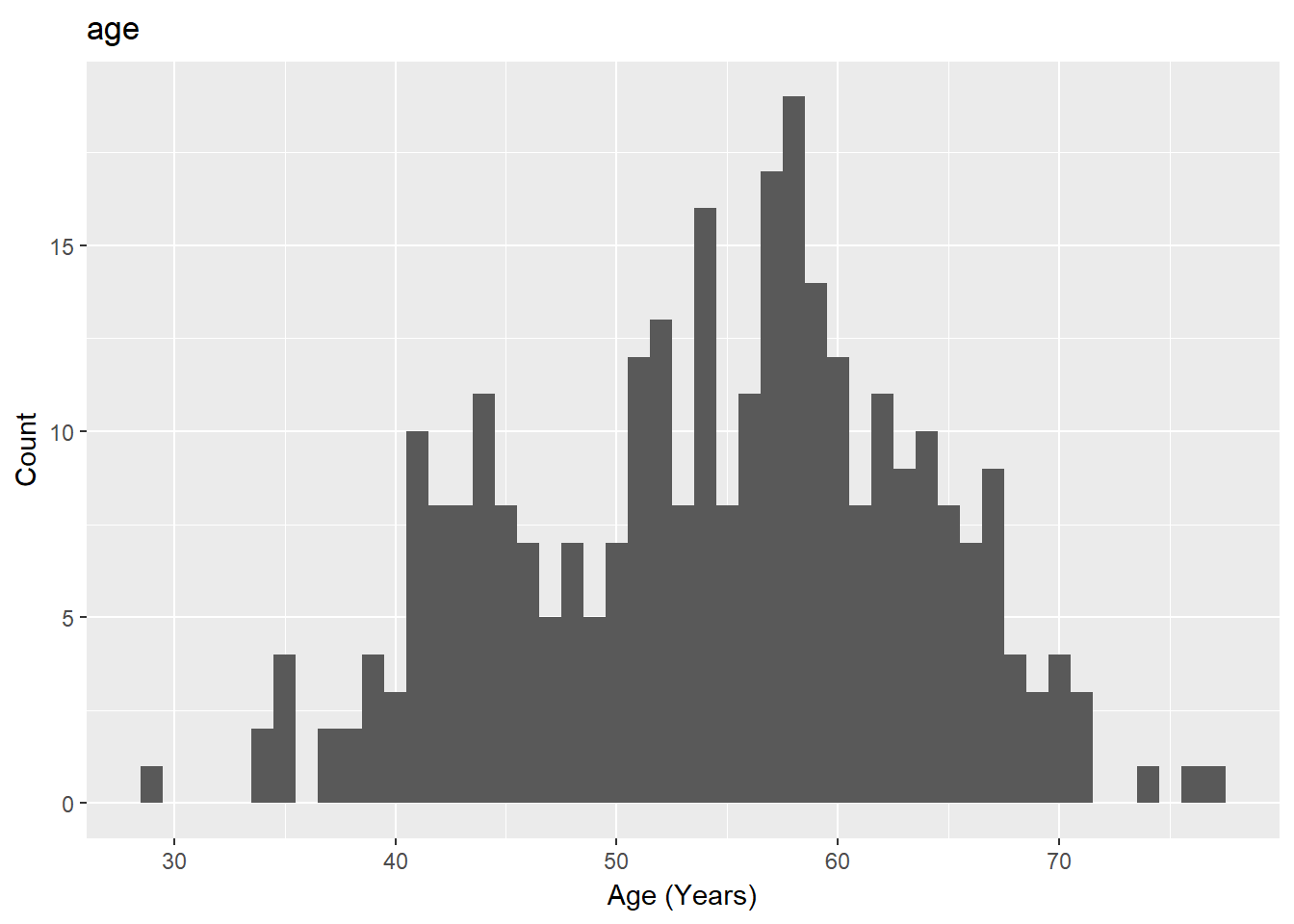Figure 1: Age Distribution

 table(heart$sex) ## ## 0 1 ## 97 206 #### cp: chest pain type + Value 1: typical angina + Value 2: atypical angina + Value 3: non-anginal pain + Value 4: asymptomatic table(heart$cp)
##
##   1   2   3   4
##  23  50  86 144

#### trestbps: resting blood pressure (in mm Hg on admission to the hospital)

summary(heart$trestbps) ## Min. 1st Qu. Median Mean 3rd Qu. Max. ## 94.0 120.0 130.0 131.7 140.0 200.0 ggplot(heart, aes(heart$trestbps)) + geom_histogram(binwidth = 1) + labs(x="Resting Blood Pressure (mm Hg)", y="Count", title="trestbps")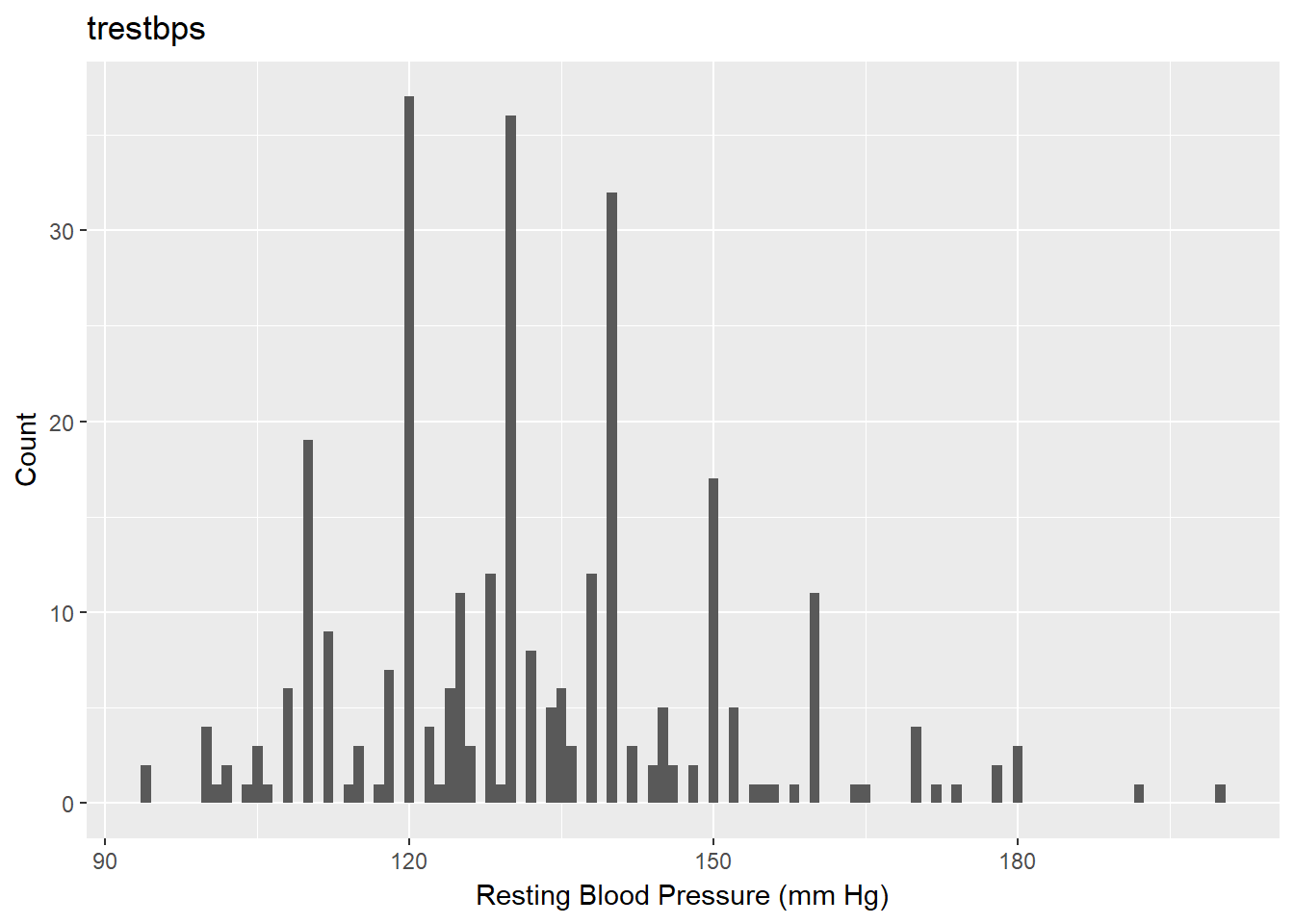Figure 2: Resting Blood Pressure Distribution

#### chol: serum cholestoral in mg/dl

summary(heart$chol) ## Min. 1st Qu. Median Mean 3rd Qu. Max. ## 126.0 211.0 241.0 246.7 275.0 564.0 ggplot(heart, aes(heart$chol)) + geom_histogram(binwidth = 1) + labs(x="Serum Cholestoral (mg/dl)", y="Count", title="chol")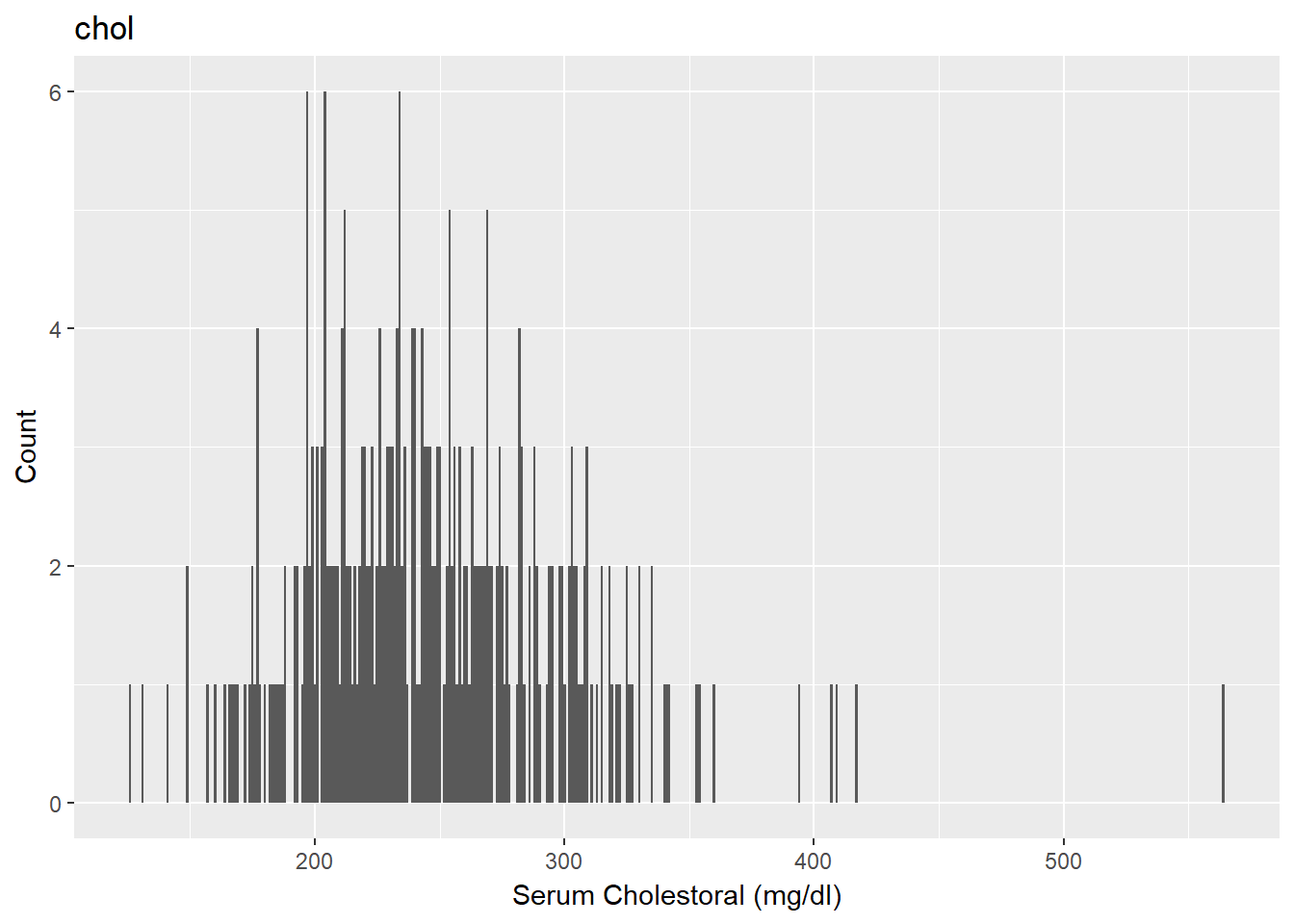Figure 3: Serum Cholestoral Distribution

table(heart$fbs) ## ## 0 1 ## 258 45 #### restecg: resting electrocardiographic results + Value 0: normal + Value 1: having ST-T wave abnormality (T wave inversions and/or ST elevation or depression of > 0.05 mV) + Value 2: showing probable or definite left ventricular hypertrophy by Estes' criteria table(heart$restecg)
##
##   0   1   2
## 151   4 148

#### thalach: maximum heart rate achieved

summary(heart$thalach) ## Min. 1st Qu. Median Mean 3rd Qu. Max. ## 71.0 133.5 153.0 149.6 166.0 202.0 ggplot(heart, aes(heart$thalach)) + geom_histogram(binwidth = 1) + labs(x="Hear Rate (b/min)", y="Count", title="thalach")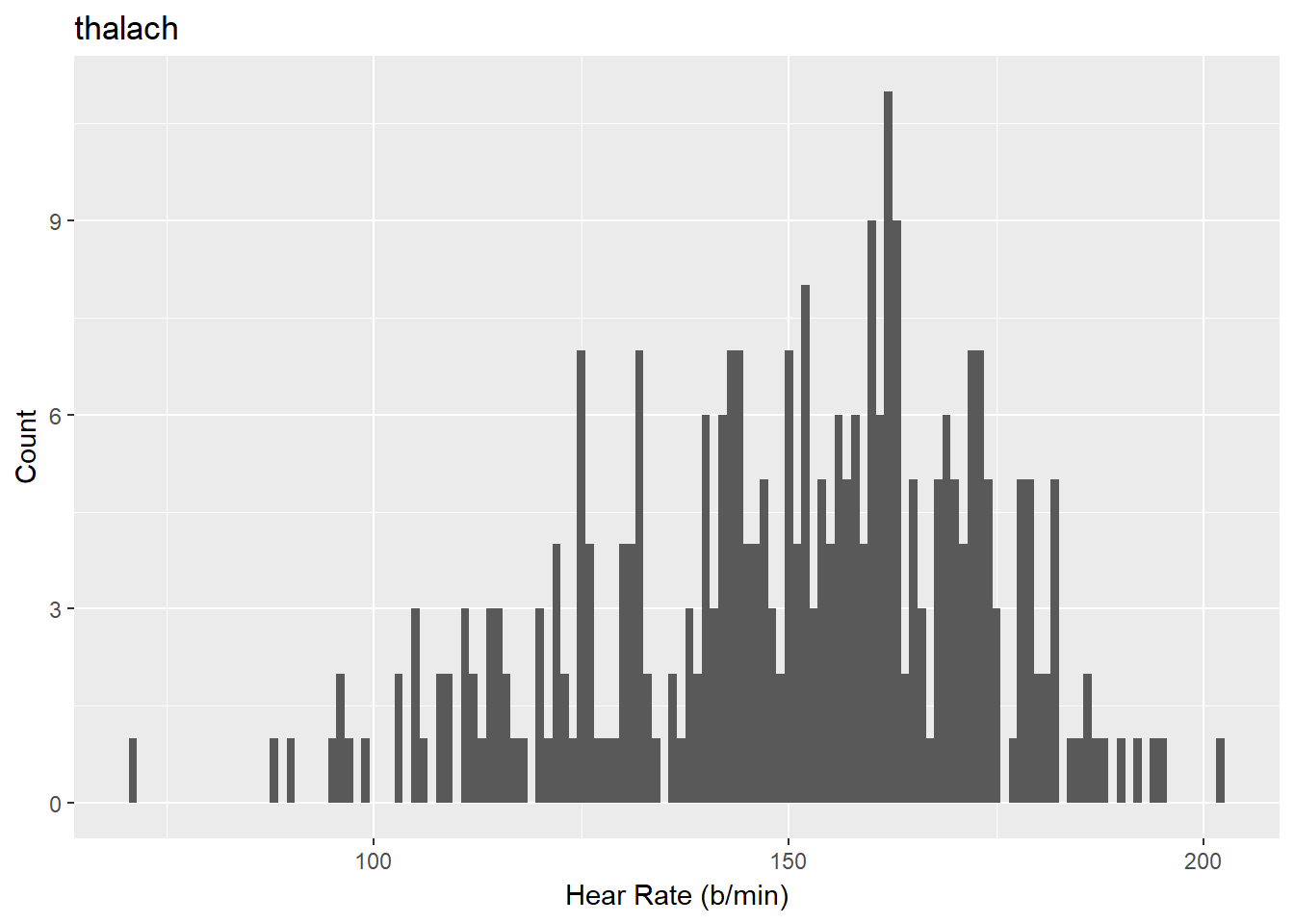Figure 4: Maximum Heart Rate Achieved Distribution

table(heart$exang) ## ## 0 1 ## 204 99 #### oldpeak = ST depression induced by exercise relative to rest summary(heart$oldpeak)
##    Min. 1st Qu.  Median    Mean 3rd Qu.    Max.
##    0.00    0.00    0.80    1.04    1.60    6.20

ggplot(heart, aes(heart$oldpeak)) + geom_histogram(binwidth = 0.1) + labs(x="ST depression (mm)", y="Count", title="oldpeak")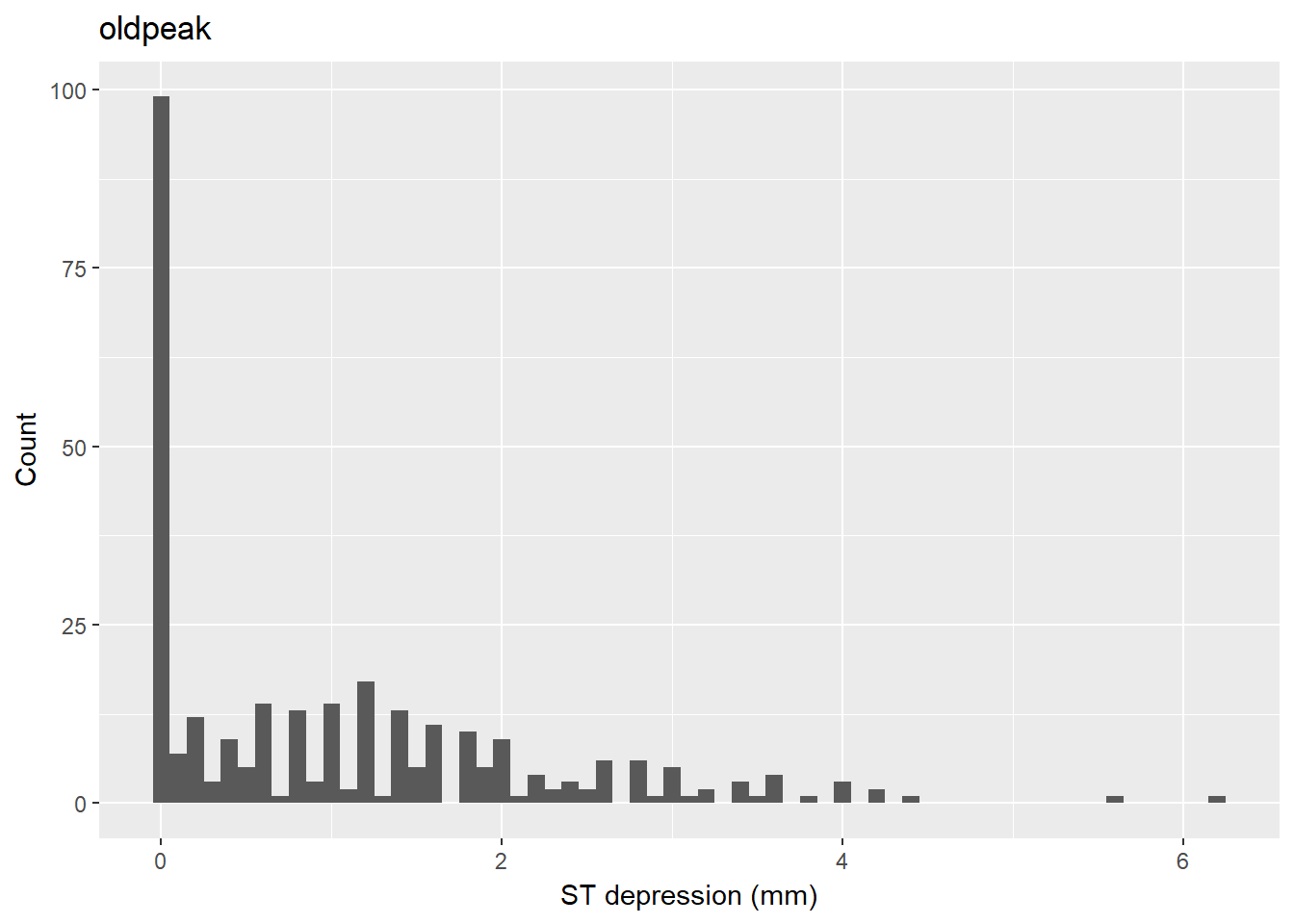Figure 5: ST Depression Induced by Exercise Relative to Rest Distribution #### slope: the slope of the peak exercise ST segment + Value 1: up sloping + Value 2: flat + Value 3: down sloping table(heart$slope)
##
##   1   2   3
## 142 140  21

table(heart$ca) ## ## ? 0.0 1.0 2.0 3.0 ## 4 176 65 38 20 #### thal: 3 = normal; 6 = fixed defect; 7 = reversible defect table(heart$thal)
##
##   ? 3.0 6.0 7.0
##   2 166  18 117

The importance of some of these variables can be defined by a domain expert, in this case a Doctor. This could help determine which variables can be omitted or how much each variable should be contributing to the classification. The data seems good to use except for some missing values.

# Data Preprocessing

### Handling Missing Values

Only categorical variables contain missing values. Imputing the missing values with the mean or median could be a good strategy. However, there are only a few so the entire rows can be removed without sacrificing too much data.

heart[heart == "?"] <- NA # Replace occurrences of '?' with 'NA'
heart <- na.omit(heart)   # Omit rows with 'NA'

diagnosis <- heart$num # Save the classification column heart <- subset(heart, select = -num) # Remove it from the data set diagnosis[diagnosis > 0] <- 1 # Set all true values to 1 ### Normalizing Data The numerical variables have different ranges which skews the results of the KNN algorithms distance calculations. Normalizing the data will prevent larger scaled variables from dominating the outcome. # Returns a normalized vector normalize <- function(x) { return ((x - min(x)) / (max(x) - min(x))) } heart$age <- normalize(heart$age) heart$trestbps <- normalize(heart$trestbps) heart$chol <- normalize(heart$chol) heart$thalach <- normalize(heart$thalach) heart$oldpeak <- normalize(heart$oldpeak) ### Dealing with Categorical Data The categorical data shouldn’t be used in a measure of distance so it must be converted to numerical data first using dummy variables. The heart disease data set has categorical data, like ‘chest pain type’, as a numerical attribute so it must be converted to a factor before the dummy.data.frame() function is run just for consistency. heart$sex <- as.factor(heart$sex) heart$cp <- as.factor(heart$cp) heart$fbs <- as.factor(heart$fbs) heart$restecg <- as.factor(heart$restecg) heart$exang <- as.factor(heart$exang) heart$slope <- as.factor(heart$slope) heart <- dummy.data.frame(heart) # Converts all factor variables into dummy variables and returns a data frame # K-Nearest Neighbor ### Splitting the Data Between Testing and Training The data is cleaned and pre-processed, it can now be used for KNN classification. To test our data, it is split between a test set and a training set. set.seed(77) # Get the same data each time idx <- sample(2, nrow(heart), replace=TRUE, prob=c(0.7, 0.3)) # Create 2 Subsets with ratio 70:30 heart_train <- heart[idx==1, ] # Training subset heart_test <- heart[idx==2, ] # Testing subset heart_train_diagnosis <- diagnosis[idx==1] # Training labels heart_test_diagnosis <- diagnosis[idx==2] # Testing labels ### Building the Classifier heart_test_predicions <- knn(train = heart_train, test = heart_test, cl = heart_train_diagnosis, k = 1) ### Evaluating Performance # Returns the percentage of correct predictions get.accuracy <- function(prediction, real) { accuracy <- prediction == real return (length(accuracy[accuracy == TRUE])/length(accuracy)) } get.accuracy(heart_test_predicions, heart_test_diagnosis) ##  0.7840909 ### Improving the Performance One way to improve KNN performance is to find the right value for K. For small data sets, looping over different values of K to find the best one is practical. # Returns the best K in range 1:max_k get.k <- function(train, test, train.cl, test.cl, max_k) { # Aggregate results k <- c() a <- c() for (i in 1:max_k){ # Run KNN prediction <- knn(train = train, test = test, cl = train.cl, k = i) # Evaluate accuracy <- get.accuracy(prediction, test.cl) # Aggregate results k <- c(k, i) a <- c(a, accuracy) } return (as.data.frame(list("K" = k, "Accuracy" = a))) } results <- get.k(heart_train, heart_test, heart_train_diagnosis, heart_test_diagnosis, (length(heart_train$age)/2))
results[results$Accuracy == max(results$Accuracy),] # Find the best K
##     K Accuracy
## 51 51    0.875
## 54 54    0.875
## 55 55    0.875
## 56 56    0.875
## 57 57    0.875
## 58 58    0.875
## 59 59    0.875
## 60 60    0.875

The best K to pick would be the lowest K with the highest accuracy. The lower K value is more efficient.

ggplot(data=results, aes(results$K, results$Accuracy)) + geom_point() + labs(x = "K", y = "Accuracy", title="How KNN Accuracy Differs with K")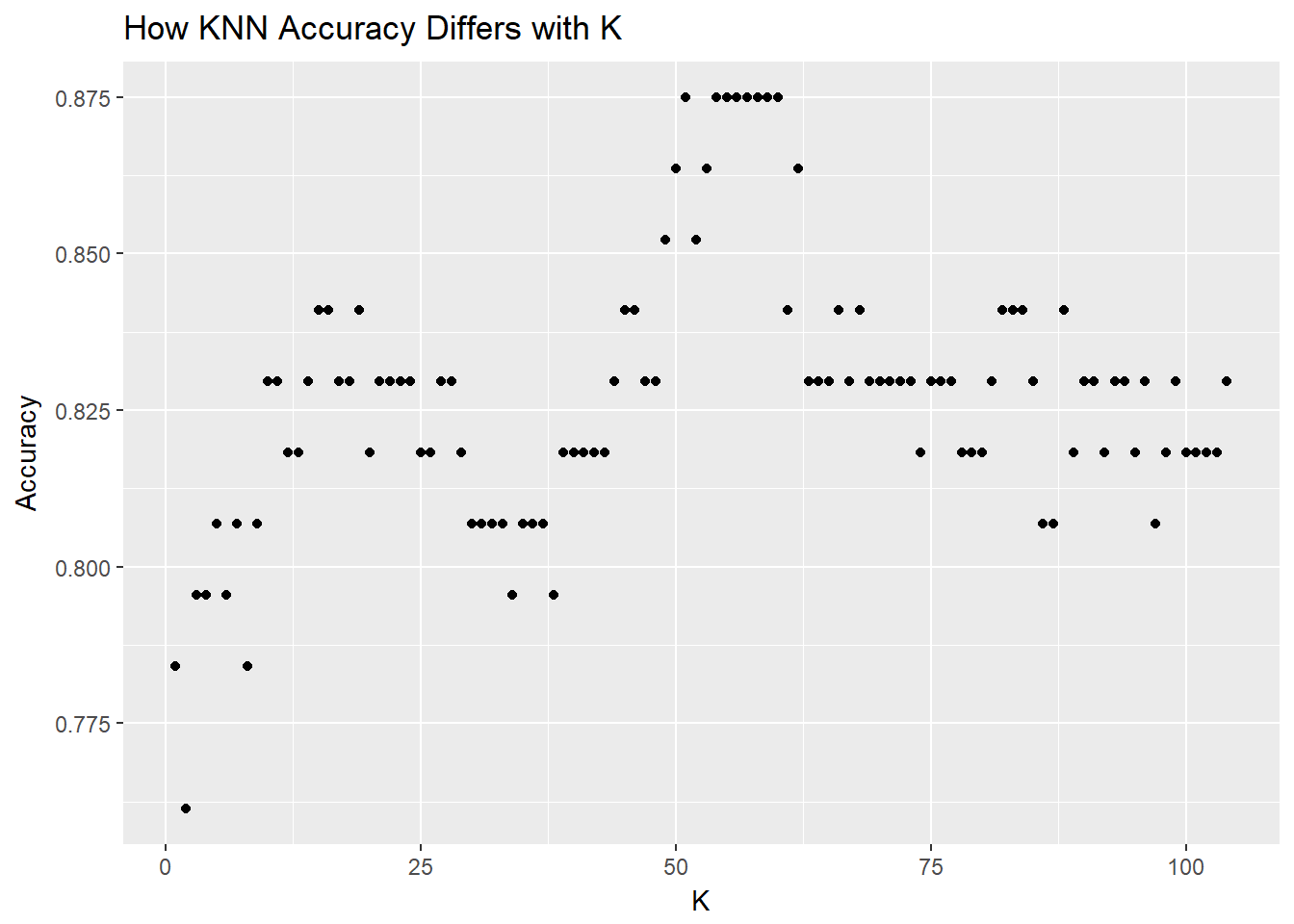Figure 6: Plot of Different K Values and Accuracy

### Cross Table

CrossTable(x = heart_test_diagnosis, y = heart_test_predicions, prop.chisq=FALSE, dnn = c('predicted', 'actual'))
##
##
##    Cell Contents
## |-------------------------|
## |                       N |
## |           N / Row Total |
## |           N / Col Total |
## |         N / Table Total |
## |-------------------------|
##
##
## Total Observations in Table:  88
##
##
##              | actual
##    predicted |         0 |         1 | Row Total |
## -------------|-----------|-----------|-----------|
##            0 |        44 |        10 |        54 |
##              |     0.815 |     0.185 |     0.614 |
##              |     0.830 |     0.286 |           |
##              |     0.500 |     0.114 |           |
## -------------|-----------|-----------|-----------|
##            1 |         9 |        25 |        34 |
##              |     0.265 |     0.735 |     0.386 |
##              |     0.170 |     0.714 |           |
##              |     0.102 |     0.284 |           |
## -------------|-----------|-----------|-----------|
## Column Total |        53 |        35 |        88 |
##              |     0.602 |     0.398 |           |
## -------------|-----------|-----------|-----------|
##
## 

# Conclusion

With very little data wrangling or preprocessing, the KNN classifier was able to correctly predict the presence of heart disease with around 87% accuracy. With good data that has been processed correctly, KNN can be utilized to classify and predict future data points. KNN is a simple but very effective algorithm.

Revision History
Revision Date Author Description
1.0 April 16, 2018 Ryan Whitell
1. Genesis